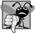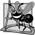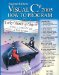# Declaring Methods with Multiple Parameters

Chapters 46 presented classes containing simple methods that had at most one parameter. Methods often require more than one piece of information to perform their tasks. We now consider how to write your own methods with multiple parameters.

The application in Fig. 7.3 and Fig. 7.4 uses a user-defined method called Maximum to determine and return the largest of three double values that are input by the user. When the application begins execution, class MaximumFinderTest's Main method (lines 610 of Fig. 7.4) creates an object of class MaximumFinder (line 8) and calls the object's DetermineMaximum method (line 9) to produce the application's output. In class MaximumFinder (Fig. 7.3), lines 1115 of method DetermineMaximum prompt the user to enter three double values and read them from the user. Line 18 calls method Maximum (declared in lines 2538) to determine the largest of the three double values passed as arguments to the method. When method Maximum returns the result to line 18, the application assigns Maximum's return value to local variable result. Then line 21 outputs the maximum value. At the end of this section, we'll discuss the use of operator + in line 21.

Figure 7.3. User-defined method Maximum.

(This item is displayed on page 279 in the print version)

 ``` 1 // Fig. 7.3: MaximumFinder.cs 2 // User-defined method Maximum. 3 using System; 4 5 public class MaximumFinder 6 { 7 // obtain three floating-point values and determine maximum value 8 public void DetermineMaximum() 9 { 10 // prompt for and input three floating-point values 11 Console.WriteLine( "Enter three floating-point values, " 12 + " pressing 'Enter' after each one: " ); 13 double number1 = Convert.ToDouble( Console.ReadLine() ); 14 double number2 = Convert.ToDouble( Console.ReadLine() ); 15 double number3 = Convert.ToDouble( Console.ReadLine() ); 16 17 // determine the maximum value 18 double result = Maximum( number1, number2, number3 ); 19 20 // display maximum value 21 Console.WriteLine( "Maximum is: " + result ); 22 } // end method DetermineMaximum 23 24 // returns the maximum of its three double parameters 25 public double Maximum( double x, double y, double z ) 26 { 27 double maximumValue = x; // assume x is the largest to start 28 29 // determine whether y is greater than maximumValue 30 if ( y > maximumValue ) 31 maximumValue = y; 32 33 // determine whether z is greater than maximumValue 34 if ( z > maximumValue ) 35 maximumValue = z; 36 37 return maximumValue; 38 } // end method Maximum 39 } // end class MaximumFinder ```

Figure 7.4. Application to test class MaximumFinder.

(This item is displayed on page 280 in the print version)

``` 1 // Fig. 7.4: MaximumFinderTest.cs
2 // Application to test class MaximumFinder.
3 public class MaximumFinderTest
4 {
5 // application starting point
6 public static void Main( string[] args )
7 {
8 MaximumFinder maximumFinder = new MaximumFinder();
9 maximumFinder.DetermineMaximum();
10 } // end Main
11 } // end class MaximumFinderTest
```

 ```Enter three floating-point values, pressing 'Enter' after each one: 3.33 2.22 1.11 Maximum is: 3.33 ```

 ```Enter three floating-point values, pressing 'Enter' after each one: 2.22 3.33 1.11 Maximum is: 3.33 ```

 ```Enter three floating-point values, pressing 'Enter' after each one: 1.11 2.22 867.5309 Maximum is: 867.5309 ```

Consider the declaration of method Maximum (lines 2538). Line 25 indicates that the method returns a double value, that the method's name is Maximum and that the method requires three double parameters (x, y and z) to accomplish its task. When a method has more than one parameter, the parameters are specified as a comma-separated list. When Maximum is called in line 18 of Fig. 7.3, the parameter x is initialized with the value of the argument number1, the parameter y is initialized with the value of the argument number2 and the parameter z is initialized with the value of the argument number3. There must be one argument in the method call for each parameter (sometimes called a formal parameter) in the method declaration. Also, each argument must be consistent with the type of the corresponding parameter. For example, a parameter of type double can receive values like 7.35 (a double), 22 (an int) or 0.03456 (a double), but not strings like "hello". Section 7.7 discusses the argument types that can be provided in a method call for each parameter of a simple type.

To determine the maximum value, we begin with the assumption that parameter x contains the largest value, so line 27 (Fig. 7.3) declares local variable maximumValue and initializes it with the value of parameter x. Of course, it is possible that parameter y or z contains the largest value, so we must compare each of these values with maximumValue. The if statement at lines 3031 determines whether y is greater than maximumValue. If so, line 31 assigns y to maximumValue. The if statement at lines 3435 determines whether z is greater than maximumValue. If so, line 35 assigns z to maximumValue. At this point, the largest of the three values resides in maximumValue, so line 37 returns that value to line 18. When program control returns to the point in the application where Maximum was called, Maximum's parameters x, y and z are no longer accessible in memory. Note that methods can return at most one value; the returned value can be a reference to an object that contains many values.

Note that result is a local variable in method DetermineMaximum because it is declared in the block that represents the method's body. Variables should be declared as fields of a class (i.e., as either instance variables or static variables of the class) only if they are required for use in more than one method of the class or if the application should save their values between calls to the class's methods.## Common Programming Error 7 1

Declaring method parameters of the same type as float x, y instead of float x, float y is a syntax errora type is required for each parameter in the parameter list.## Software Engineering Observation 7 4

A method that has many parameters may be performing too many tasks. Consider dividing the method into smaller methods that perform the separate tasks. As a guideline, try to fit the method header on one line if possible.

Implementing Method Maximum by Reusing Method Math.Max

Recall from Fig. 7.2 that class Math has a Max method that can determine the larger of two values. The entire body of our maximum method could also be implemented with nested calls to Math.Max, as follows:

```return Math.Max( x, Math.Max( y, z ) );
```

The leftmost call to Math.Max specifies arguments x and Math.Max( y, z ). Before any method can be called, all its arguments must be evaluated to determine their values. If an argument is a method call, the method call must be performed to determine its return value. So, in the preceding statement, Math.Max( y, z ) is evaluated first to determine the maximum of y and z. Then the result is passed as the second argument to the other call to Math.Max, which returns the larger of its two arguments. Using Math.Max in this manner is a good example of software reusewe find the largest of three values by reusing Math.Max, which finds the larger of two values. Note how concise this code is compared to lines 2737 of Fig. 7.3.

Assembling Strings with String Concatenation

C# allows string objects to be created by assembling smaller strings into larger strings using operator + (or the compound assignment operator +=). This is known as string concatenation. When both operands of operator + are string objects, operator + creates a new string object in which a copy of the characters of the right operand are placed at the end of a copy of the characters in the left operand. For example, the expression "hello " + "there" creates the string "hello there" without disturbing the original strings.

In line 21 of Fig. 7.3, the expression "Maximum is: " + result uses operator + with operands of types string and double. Every value of a simple type in C# has a string representation. When one of the + operator's operands is a string, the other is implicitly converted to a string, then the two are concatenated. In line 21, the double value is implicitly converted to its string representation and placed at the end of the string "Maximum is: ". If there are any trailing zeros in a double value, these will be discarded when the number is converted to a string. Thus, the number 9.3500 would be represented as 9.35 in the resulting string.

For values of simple types used in string concatenation, the values are converted to strings. If a boolean is concatenated with a string, the bool is converted to the string "True" or "False" (note that each is capitalized). All objects have a ToString method that returns a string representation of the object. When an object is concatenated with a string, the object's ToString method is implicitly called to obtain the string representation of the object.

Line 21 of Fig. 7.3 could also be written using string formatting as

```Console.WriteLine( "Maximum is: {0}", result );
```

As with string concatenation, using a format item to substitute an object into a string implicitly calls the object's ToString method to obtain the object's string representation. You will learn more about method ToString in Chapter 8, Arrays.

When a large string literal is typed into an application's source code, you can break that string into several smaller strings and place them on multiple lines for readability. The strings can be reassembled using either string concatenation or string formatting. We discuss the details of strings in Chapter 16, Strings, Characters and Regular Expressions.## Common Programming Error 7 2

It is a syntax error to break a string literal across multiple lines in an application. If a string does not fit on one line, split the string into several smaller strings and use concatenation to form the desired string.## Common Programming Error 7 3

Confusing the + operator used for string concatenation with the + operator used for addition can lead to strange results. The + operator is left-associative. For example, if integer variable y has the value 5, the expression "y + 2 = " + y + 2 results in the string "y + 2 = 52", not "y + 2 = 7", because first the value of y (5) is concatenated with the string "y + 2 = ", then the value 2 is concatenated with the new larger string "y + 2 = 5". The expression "y + 2 = " + (y + 2) produces the desired result "y + 2 = 7".

### Notes on Declaring and Using MethodsVisual C# 2005 How to Program (2nd Edition)
ISBN: 0131525239
EAN: 2147483647
Year: 2004
Pages: 600